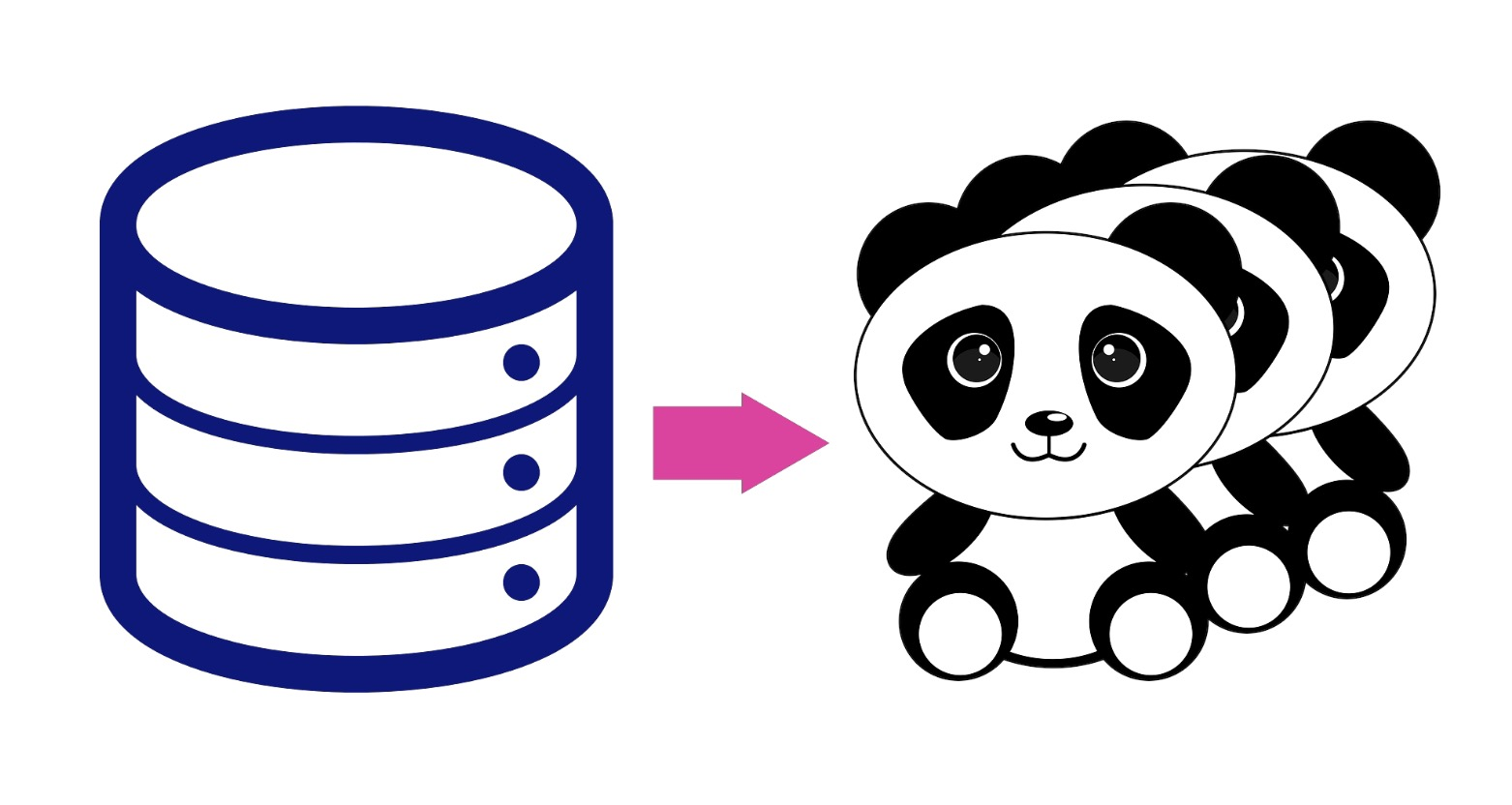# Pandas versus SQL Comparison : JOINSQL (Structured Query Language) and Pandas (Python library built on top Numpy package) are widely used by data scientists, because these programming languages enable them to read, manipulate, write and retrieve data (most of the time stored in a database or data warehouses such as BigQuery or Amazon RedShift). Every data scientist is aware that her/his job will include data extraction and cleaning, therefore knowing SQL or Pandas is often required.

In this article, we will give you a simple comparison of those important tools for data scientists with a focus on the JOIN function (used to combine from two or more tables or dataframes).

Let's go over the different JOINs available in SQL:

1. INNER JOIN: Returns records that have matching values in both tables

2. LEFT JOIN: ​Returns all records from the left table, and the matched records from the right table

3. RIGHT JOIN: Returns all records from the right table, and the matched records from the left table

4. FULL JOIN: Returns all records when there is a match in either left or right table

Let's dive in and now learn how to join two tables or data frames using SQL and Pandas

1. INNER JOIN

SQL

``SELECT * FROM table1 INNER JOIN table2     ON table1.key = table2.key;``

Pandas

``````import pandas as pd
#df1 and df2 are two pandas dataframespd.merge(df1, df2, on='key') ``````

2. LEFT JOIN

SQL

``SELECT * FROM table1 LEFT JOIN table2     ON table1.key = table2.key;``

Pandas

``````import pandas as pd
#df1 and df2 are two pandas dataframespd.merge(df1, df2, on='key',how='left') ``````

3. RIGHT JOIN

SQL

``SELECT * FROM table1 RIGHT JOIN table2     ON table1.key = table2.key;``

Pandas

``````import pandas as pd
#df1 and df2 are two pandas dataframespd.merge(df1, df2, on='key',how='right') ``````

4. FULL JOIN

SQL

``SELECT * FROM table1 FULL JOIN table2     ON table1.key = table2.key;``

Pandas

``````import pandas as pd
#df1 and df2 are two pandas dataframespd.merge(df1, df2, on='key',how='outer') ``````

We hope that you will use those snippets to join some tables or data frames in your daily job!